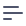# Segments

Segments are predefined filters.

You can use segments to define complex filtering logic in SQL. For example, users for one particular city can be treated as a segment.

cube(Users, {
// ...

segments: {
sfUsers: {
sql: ${CUBE}.location = 'San Francisco' } } });  Or use segments to implement cross-column OR logic: cube(Users, { // ... segments: { sfUsers: { sql: ${CUBE}.location = 'San Francisco' or ${CUBE}.state = 'CA' } } });  As with other cube member definitions segments can be generated: const userSegments = { sfUsers: ['San Francisco', 'CA'], nyUsers: ['New York City', 'NY'] } cube(Users, { // ... segments: { ...(Object.keys(userSegments).map(segment => ({ [segment]: { sql: ${CUBE}.location = '${userSegments[segment]}' or${CUBE}.state = '${userSegments[segment]}' } })).reduce((a, b) => ({ ...a, ...b }))) } });  After defining a segment, you can pass it in query object: { measures: ['Users.count'], segments: ['Users.sfUsers'] }  ## Segments vs Dimension Filters As segments are just predefined filters there's a question when to use segments instead of dimension filters. Let's consider an example: cube(Users, { // ... dimensions: { location: { sql: location, type: string } }, segments: { sfUsers: { sql: ${CUBE}.location = 'San Francisco'
}
}
});


In this case following queries are equivalent:

{
measures: ['Users.count'],
filters: [{
member: 'Users.location',
operator: 'equals',
values: ['San Francisco']
}]
}


and

{
measures: ['Users.count'],
segments: ['Users.sfUsers']
}


This case is a bad candidate for segment usage and dimension filter works better here. Users.location filter value can change a lot for user queries and Users.sfUsers segment won't be used much in this case.

Good candidate case for segment is when you have complex filtering expression which can be reused for a lot of user queries. For example:

cube(Users, {
// ...

segments: {
sfNyUsers: {
sql: ${CUBE}.location = 'San Francisco' OR${CUBE}.location like '%New York%'
}
}
});